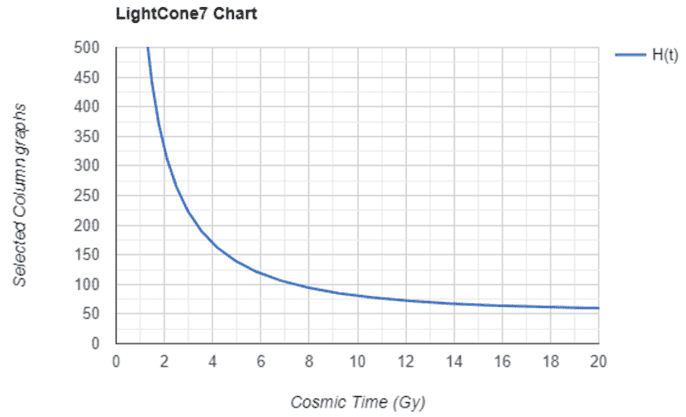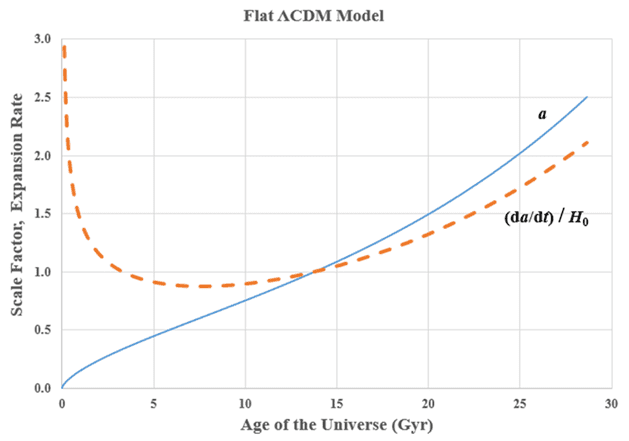# Question about the Hubble Constant

• I
Thecla
TL;DR Summary
does the Hubble Constant change over time?
If there were humans on Earth 2.5 billion years ago (universe 20% younger) and they had today's equipment , would they measure the Hubble constant smaller, larger or the same?

Larger. It was always larger in the past, and will be always smaller towards the future.

•Halc
Gold Member
It indeed will always be smaller in the future, but not arbitrarily smaller. It was arbitrarily higher in the past, especially in the first fraction of a second of the big bang.

I think I read that it is predicted to settle down to about 57 km/sec/mpc and go no lower. That's exponential expansion as opposed to the more linear expansion in the (fairly) recent past.
Of course if it continues to fall always, you end up with a big-rip finale.

Of course if it continues to fall always, you end up with a big-rip finale.
Big rip is when it starts growing without bound, surely.

Gold Member
Big rip is when it starts growing without bound, surely.
Duh, of course. Decreasing without bound happens with no expansion, such as the Milne solution.
Brain fart. Sorry.

Last edited:
•jim mcnamara and Bandersnatch
Well, I might have clicked like too early ;)
You meant Milne solution? The one with no acceleration/deceleration (but with expansion)? The one where H decreases towards 0 (i.e. not without bound)?
Decreasing without bound leads to reversed expansion. I.e. contraction. A big crunch.

Staff Emeritus
Gold Member
Summary:: does the Hubble Constant change over time?

If there were humans on Earth 2.5 billion years ago (universe 20% younger) and they had today's equipment , would they measure the Hubble constant smaller, larger or the same?

To a good approximation, our universe presently is a (spatially) flat FLRW universe that consists of matter (##w=0##) and dark energy (##w=-1##). The scale factor for such a universe is
$$a\left(t\right) = A \sinh^{\frac{2}{3}} \left(Bt\right),$$
where ##A## and ##B## are constants. The Hubble parameter is
$$H = \frac{\frac{da}{dt}}{a}.$$
Can you use this to find and expression for the Hubble parameter? If not, I will (eventually) fill in the details

Mentor
I think I read that it is predicted to settle down to about 57 km/sec/mpc and go no lower. That's exponential expansion
Yes.

as opposed to the more linear expansion in the (fairly) recent past.
The expansion was never linear. Before a few billion years ago, it was more or less parabolic (matter dominated, density near critical).

Of course if it continues to fall always, you end up with a big-rip finale.
No. If it continues to fall always, asymptoting towards zero, that would be a matter dominated universe with density less than or equal to critical and zero cosmological constant. That was the "best fit" model cosmologists were using until the late 1990s when the accelerating expansion was discovered.

If it continues to fall always and becomes negative, that would be a matter dominated universe with density greater than critical and zero cosmological constant--i.e., a closed universe that will recollapse to a "big crunch".

Decreasing without bound happens with no expansion, such as the Milner solution.
The Milne universe does have expansion; even though it is flat Minkowski spacetime in funny coordinates, the congruence of "comoving" observers in this universe has positive expansion. This universe is the limiting case of an "open" universe (density less than critical) when the density goes to zero.

Gold Member
The Milne universe does have expansion; even though it is flat Minkowski spacetime in funny coordinates
Yes, but the expansion rate, expressed in km/sec/mpc, drops to arbitrarily low over time, even if never to zero. The Hubble constant is never a constant, at least not yet.

Mentor
the expansion rate, expressed in km/sec/mpc, drops to arbitrarily low over time, even if never to zero.
Yes, that follows from the fact that this is a limiting case of an open universe.

Thecla
I don't understand. If it were larger in the past, why do astronomers say that the expansion is accelerating. Shouldn't this mean the Hubble constant is increasing?

Mentor
If it were larger in the past, why do astronomers say that the expansion is accelerating. Shouldn't this mean the Hubble constant is increasing?
When astronomers talk about the expansion accelerating, they are talking about the rate of change of the scale factor with respect to time, i.e., ##\dot{a}##. "Accelerating" means ##\dot{a}## is increasing. But the Hubble constant is ##\dot{a} / a##, and that is decreasing. For the case that @George Jones gave the equation for in post #7, you should be able to calculate explicitly both of these quantities and confirm that what I have just said is true.

Gold Member
Summary:: does the Hubble Constant change over time?

If there were humans on Earth 2.5 billion years ago (universe 20% younger) and they had today's equipment , would they measure the Hubble constant smaller, larger or the same?

Here is a H vs t plot:Please see the thread, The Hubble constant − constant in what way?

Gold Member
When astronomers talk about the expansion accelerating, they are talking about the rate of change of the scale factor with respect to time, i.e., ##\dot{a}##. "Accelerating" means ##\dot{a}## is increasing. But the Hubble constant is ##\dot{a} / a##, and that is decreasing. For the case that @George Jones gave the equation for in post #7, you should be able to calculate explicitly both of these quantities and confirm that what I have just said is true.
Another way to put this is the accelerated expansion means that far-away galaxies are moving away from one another at speeds that increase with time.

A constant expansion rate is one way to cause this to happen: constant rate means constant recession velocity over distance ratio. So increase the distance, increase the recession velocity.

As long as the rate of expansion (the Hubble parameter) is decreasing slowly enough, recession velocities will increase as things move away from one another. This is the case today. I'm the far future, if the dark energy behaves like a cosmological constant, the rate of expansion will become nearly constant.

•Ibix
Gold Member
When astronomers talk about the expansion accelerating, they are talking about the rate of change of the scale factor with respect to time, i.e., ##\dot{a}##. "Accelerating" means ##\dot{a}## is increasing. But the Hubble constant is ##\dot{a} / a##, and that is decreasing. For the case that @George Jones gave the equation for in post #7, you should be able to calculate explicitly both of these quantities and confirm that what I have just said is true.

Using Jorrie’s calculator, we can calculate a(t) and the Hubble parameter, H(t). The expansion rate can be calculated with the equation,

da/dt = a(t) H(t) (see Post #7)​

The results are plotted below:The above figure suggests that, according to the ΛCDM model, the expansion of the universe is accelerating.